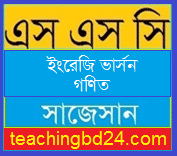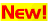# EV Mathematics Suggestion and Question Patterns of SSC Examination 2019

EV Mathematics Suggestion and Question Patterns of SSC Examination 2019. Mathematics is originated from the process of expressing quantities in symbols or numbers. The history of numbers is as ancient as the history of human civilization. Greek Philosopher Aristotle According to the formal inauguration of mathematics occurs in the practice of mathematics by the sect of the priest in ancient Egypt. So, the number based mathematics is the creation of about two thousand years before the birth of Christ. After that, moving from many nations and civilization, numbers and principles of numbers have gained a universal form at present.

## EV Mathematics Suggestion and Question Patterns of SSC Examination 2019## SSC Mathematics MCQ Question With Answer 2020EV Mathematics Model Question

Model Question No. 1

Model Question No. 2

Model Question No. 3

Model Question No. 4

Model Question No. 5

Model Question No. 6

Model Question No. 7

Model Question No. 8

Model Question No. 9

Model Question No. 10

Model Question No. 11

Model Question No. 12

Model Question No. 13

Model Question No. 14

Model Question No. 15

Model Question No. 16

The mathematicians in India first introduce zero (0) and 10 based place value system for counting natural numbers, which is considered a milestone in describing numbers. Chinese and Indian mathematicians extended the idea zero, real numbers, negative number, integer and fractional numbers which the Arabian mathematicians accepted in the middle age. But the credit of expressing number through decimal fraction is awarded to the Muslim Mathematicians. Again they introduce first the irrational numbers in square root form as a solution of the quadratic equation in algebra in the 11th the century. According to the historians, very near to 50 BC the Greek Philosophers also felt the necessity of irrational number for drawing geometric figures, especially for the square root of 2.

teachingbd24.com is such a website where you will get all kinds of necessary information regarding educational notes, suggestions and question patterns of schools, colleges, and madrasas. Particularly, you will get here special notes of physics that will be immensely useful to both students and teachers. The builder of the website is Mr. Md. Shah Jamal who has been serving for 32 years as an Assistant Professor of Physics at BAF Shaheen College Dhaka. He expects that this website will meet up all the needs of Bengali version learners /students. He has requested concerned students and teachers to spread this website home and abroad.

এখানে ক্লিক করে ২০১৯ সালের বিভিন্ন বিষয়ের সাজেসান ও প্রশ্নের ধরণ জেনে নিন।